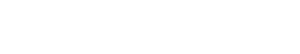# Topic word probabilities

Topic word probabilities, also called topic-specific word probabilities, are a type of topic model where words are assigned probabilities according to the topics they represent. They are a type of statistical models that are used to discover the hidden thematic structures in a collection of documents. Topic models can be used for tasks such as document classification, information retrieval, and recommendation systems.

The most popular topic model is Latent Dirichlet Allocation (LDA). LDA is a generative statistical model that allows us to discover the latent topics that exist in a collection of documents. In LDA, each document is represented as a distribution of topics, and each topic is represented as a distribution of words.

Topic word probabilities are a type of topic model where words are assigned probabilities according to the topics they represent. Topic word probabilities can be used to calculate the probability of a word belonging to a particular topic. This information can be used to improve document classification and information retrieval.

Topic word probabilities can be compared to other similar terms, such as conditional probability and joint probability. Conditional probability is the probability of an event occurring given that another event has occurred. Joint probability is the probability of two events occurring at the same time.

While topic models are a type of statistical model, topic word probabilities are not always statistically significant. However, they can still be useful for tasks such as document classification and information retrieval.

## How to calculate topic word probabilities :

Topic word probabilities can be calculated using a topic model. To calculate the topic word probability for a given word, we need to first determine the topic that the word belongs to. We can do this by looking at the distribution of topics for the document that the word is in. Once we have determined the topic that the word belongs to, we can then calculate the probability of the word belonging to that topic.

For example, let’s say we have a document with two topics, Topic A and Topic B. We can use a topic model to discover that Topic A is represented by 30% of the words in the document and Topic B is represented by 70% of the words in the document. If we then look at the distribution of words for Topic A, we might find that the word “the” has a probability of 0.3. This means that there is a 30% chance that the word “the” belongs to Topic A.Unlock the power of actionable insights with AI-based natural language processing.